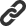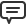# Blog

All Blog Posts  |  Next Post  |  Previous Post

#Improvements for symbolic expressions simplification in TMS Analytics & Physics developing library

Bookmarks:

#### Wednesday, December 20, 2017

One of the unique features of the Analytics library is symbolic derivatives calculation. The derivative can be calculated just with several lines of Delphi code, for an example:

```var
f, df: string;
t: TTranslator;
begin
t:= TTranslator.Create;
f:= 'x^2*e^(x/3)';
df:= t.Derivative(f, 'x');
//...
end;```

The Analytics library guarantees that the result derivative expression is syntactically and mathematically correct and satisfies the derivative rules. From the first version, the derivative algorithm automatically simplifies the result derivative expression. Nevertheless, the library is not a Computer Algebra system, so it cannot simplify all the result expressions to the optimal representation.

New version 2.3 introduces new simplification algorithms for providing more readable and short symbolic derivatives. New simplification algorithms include: constants reducing in sum and product expressions; reducing nested sum or product expressions to one; reducing negation pairs in product expressions and others.

There are some examples of functions and their derivative expressions, evaluated with the old version of the library and with the 2.3 version:

```f(x)       : x^2*e^(x/3)
df/dx (old): (2*x)*e^(x/3)+(e^(x/3)*(1/3))*x^2
df/dx (2.3): 2*x*e^(x/3)+e^(x/3)/3*x^2

f(x)       : 2*x^3+A/4*x^2-x/3
df/dx (old): (3*x^2)*2+((2*x)*A)/4-1/3
df/dx (2.3): 6*x^2+1/2*x*A-1/3

f(x)       : (A/2)*arctan(x^2)
df/dx (old): ((1/(1+(x^2)^2))*(2*x))*(A/2)
df/dx (2.3): x*A/(1+x^4)

f(x)       : P{n-1 m+2}(x)
df/dx (old): ((((n-1)+1)-(m+2))*P{(n-1)+1 m+2}(x) -(((n-1)+1)*x)*P{n-1 m+2}(x))/(x^2-1)
df/dx (2.3): ((n-m-2)*P{n m+2}(x)-n*x*P{n-1 m+2}(x))/(x^2-1)

```

As can be seen from the examples, new version gives shorter and more readable symbolic expressions for different function cases. The simplified representation of the formulae also reduces the calculation time. The new library version is already available here

Bruno Fierens

Bookmarks: